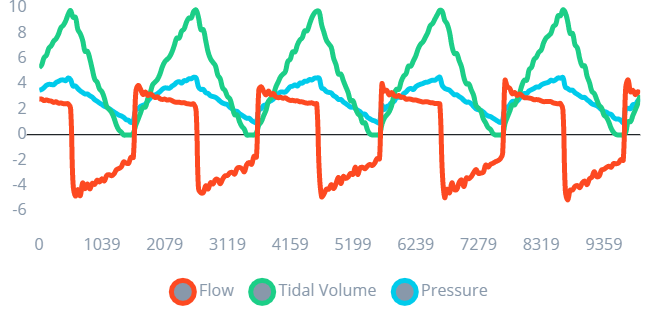# The Hardware (ESP32) Prediction Isn't As Accurate As It Was In Model Development

Hi Edge Impulse Team,

I’m aiming to classify ventilator waveforms in near real time. As shown in the picture, I’m using an ESP32 with flow (SFM3300) and pressure (MPX5010DP) sensors.

I’m using a data forwarder to record three metrics at 50 Hz: flow, volume, and pressure. The dataset was made up of eight (8) labels, each lasting 10 minutes. The waveform graph example is presented below.The following settings for the Impulse Design are shown below.
Window size: 1500 ms
Window Increase: 250 ms
Features Extraction: Spectral Analysis

The results of the neural network once it has been configured are provided below.
Training
Model Loss

Model Accuracy

Confusion Matrix
Validation

Testing

The model appears to fit and perform well in the live classification. However, when the model is run on hardware, it fails to categorize the ventilator waveform, data from sensors.
Below is a sample sketch.

void loop() {
unsigned int rawData = sensirionFlow.getValue();

// Features buffer full? Then classify!
if (feature_ix == EI_CLASSIFIER_DSP_INPUT_FRAME_SIZE) {
ei_impulse_result_t result;

// Create signal from features frame
signal_t signal;
numpy::signal_from_buffer(features, EI_CLASSIFIER_DSP_INPUT_FRAME_SIZE, &signal);

// Run classifier
EI_IMPULSE_ERROR res = run_classifier(&signal, &result, false);
if (res != 0) return;

Serial.print(F("Predictions (DSP: “));
Serial.print(result.timing.dsp);
Serial.print(F(” ms., Classification: “));
Serial.print(result.timing.classification);
Serial.print(F(” ms., Anomaly: "));
Serial.print(result.timing.anomaly);
Serial.print(“ms.): \n”);

// Print the predictions
for (size_t ix = 0; ix < EI_CLASSIFIER_LABEL_COUNT; ix++) {
Serial.print(result.classification[ix].label);
Serial.print(F(":\t"));
Serial.print(result.classification[ix].value);
Serial.print(F("\n"));
}

#if EI_CLASSIFIER_HAS_ANOMALY == 1
Serial.print(F(“anomaly:\t”));
Serial.print(result.anomaly);
Serial.print(F("\n"));
#endif

// Reset features frame
feature_ix = 0;
}
}

{
(void) pvParameters;
TickType_t xLastWakeTime;
const TickType_t xFrequency = FREQUENCY_HZ;
for (;{
// Scaled and filtered the waveform for plotting
filteredFlow = flowFilter.get() - 0.00;
scaledVol = tidalVolume / 25;
filteredPres = pressureFilter.get() - 1.02F;

// Fill the features buffer
features[feature_ix++] = filteredFlow;
features[feature_ix++] = scaledVol;
features[feature_ix++] = filteredPres;

}
}

Is the model underfit / overfit? or there are any adjustment or recommendation for this one? Thank you so much for your response.

Hello @TronixLabPH,

Just had a look at your project, it looks fine on the studio sideAbout your embedded code, I’m not 100% sure that you fill the buffer at the same frequency as the one you use for your data collection (52Hz)…

If you have a look at how we fill the buffer in the nano_ble33_sense_accelerometer.ino example (provided when downloading the Arduino Library from the studio), here is how it is done:

``````// Allocate a buffer here for the values we'll read from the IMU
float buffer[EI_CLASSIFIER_DSP_INPUT_FRAME_SIZE] = { 0 };

for (size_t ix = 0; ix < EI_CLASSIFIER_DSP_INPUT_FRAME_SIZE; ix += 3) {
// Determine the next tick (and then sleep later)
uint64_t next_tick = micros() + (EI_CLASSIFIER_INTERVAL_MS * 1000);

IMU.readAcceleration(buffer[ix], buffer[ix + 1], buffer[ix + 2]);

buffer[ix + 0] *= CONVERT_G_TO_MS2;
buffer[ix + 1] *= CONVERT_G_TO_MS2;
buffer[ix + 2] *= CONVERT_G_TO_MS2;

delayMicroseconds(next_tick - micros());
}
``````

Is your INTERVAL_MS the same as EI_CLASSIFIER_INTERVAL_MS ?

Also, are the “processing” or “calibration” of your values done the same way as during your data collection?

``````filteredFlow = flowFilter.get() - 0.00;
scaledVol = tidalVolume / 25;
filteredPres = pressureFilter.get() - 1.02F;
``````

Let me knowRegards,

Louis

Thank you Louis for your response, I’m glad to hear from you. Refer to the data forwarder documentation, the INTERVAL_MS is defined by

``````#define FREQUENCY_HZ        50
#define INTERVAL_MS         (1000 / (FREQUENCY_HZ + 1))
``````

The sampling rate given by FREQUENCY_HZ , and the data forwarder detected a frequency of 52 Hz. Yes, I did the same thing with my data forwarder sketch

filteredFlow = flowFilter.get() - 0.00;
scaledVol = tidalVolume / 25;
filteredPres = pressureFilter.get() - 1.02F;

I have following new questions:

1. Is the EI_CLASSIFIER_INTERVAL_MS already defined by Edge Impulse Arduino library?
2. Is the sketch structure in the library examples (nano_ble33_sense_accelerometer_continues) compatible with ESP32 architecture? If yes, I will try to modify it according to the sensors I used.

Hello @TronixLabPH,

It is more or less compatibleI mean it should be compatible but not optimized. Better to use the RTOS scheduler vTaskDelay as you did for example. But to begin with, I’d follow the example and adapt it with your sensors to make sure you’ve got good results.

And for the EI_CLASSIFIER_INTERVAL_MS, it actually comes from the model-parameters/model_metadata.h included when you download EI models from the studio.

Regards,

Louis

Hi @louis

Thanks for the feedback. Aligning the sample rate did not solve the problem, so I increased the model’s window size and it now works well.

3 Likes